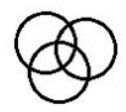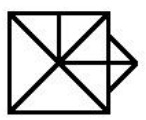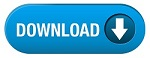# SSC CHSL (Tier - 1) Online Exam Paper - 2020 "held on 12 August 2021" Evening-Shift (General Intelligence)## Evening-Shift (General Intelligence)

Q.1 Select the number that can replace the question mark (?) in the following series.

25, 26, 53, 160, 641, ?

1. 3403

2. 3202

3. 3206

4. 2908

Q.2 Select the option figure which contains figure X embedded in it as its part (rotation is NOT allowed).Q.3 ‘East’ is related to ‘Direction’ in the same way as ‘Summer’ is related to ‘______’.

1. Room Cooler

2. Temperature

3. Season

4. Heat

Q.4 Select the option in which the numbers in the set share the same relationship as that shared by the numbers of the given set.

(14, 18, 42)

1. (40, 23, 94)

2. (20, 15, 100)

3. (22, 18, 74)

4. (16, 21, 56)

Q.5 Select the option in which the numbers are related in the same way as are the numbers of the following set.

(2, 3, 62)

1. (8, 5, 225)

2. (5, 9, 288)

3. (6, 4, 122)

4. (7, 4, 226)

Q.6 If 'A & B' means 'A is the father of B' and 'A @ B' means 'A is the sister of B', then which of the following expressions means 'H is the father of M'?

1. K & H @ P @ M

2. H @ K @ P & M

3. H & K @ P @ M

4. M & K @ P @ H

Q.7 In a certain code language, 'FROZEN' is coded as '504' and 'TONSILS' is coded as '756'. How will 'MARINE' be coded in that language?

1. 360

2. 652

3. 520

4. 456

Q.8 Select the set of classes the relationship among which is best illustrated by the following Venn diagram.1. Owls, Mammals, Reptiles

2. Doctors, Fathers, Brothers

3. Mothers, Engineers, Women

4. Stationery, Pens, Pencils

Q.9 Select the combination of letters that when sequentially placed in the blanks of the given series will complete the series.

c k _ g k c _ t _ k _ k t g _ c k _ g k

1. t, k, c, g, k, t

2. t, k, g, c, c, t

3. t, g, k, c, k, t

4. t, k, g, c, k, t

Q.10 Select the option that is related to the fourth number in the same way as the first number is related to the second number and the fifth number is related to the sixth number.

127 : 11 :: ? : 16 :: 367 : 19

1. 248

2. 216

3. 262

4. 280

Q.11 A piece of paper is folded and cut as shown below in the question figures. Select form the option figures, how it will appear when unfolded.Q.12 Select the correct combination of mathematical signs to sequentially replace the * signs to balance the following equation.

40 * 15 * 3 * 72 * 9 = 77

1. +, ÷, −, ×, =

2. ×, ÷, +, −, =

3. +, ×, ÷, −, =

4. +, ×, −, ÷, =

Q.13 In a code language, TOUCH is written as UTOHC. How will PLANT be written in that language?

1. TPANM

2. ALRPT

3. TPNLA

4. QMBOU

Q.14 Three of the following four words are alike in a certain way and one is different. Select the odd one.

1. Lungs

2. Liver

3. Heart

4. Nose

Q.15 Four number-pairs have been given, out of which three are alike in some manner and one is different. Select the number-pair that is different.

1. 19 : 324

2. 5 : 35

3. 13 : 195

4. 7 : 63

Q.16 How many triangles are there in the given figure?1. 15

2. 16

3. 17

4. 14

Q.17 Select the correct mirror of the given figure when a mirror is placed on the right side of the figure.Q.18 Select the correct option that indicates the arrangement of the given words in the order in which they appear in an English dictionary.

1. Industry

2. Indent

3. Indoor

4. Indolent

5. Index

1. 2, 4, 5, 3, 1

2. 2, 1, 3, 5, 4

3. 1, 2, 3, 5, 4

4. 2, 5, 4, 3, 1

Q.19 In a class of 68 students, 34 students participated only in Debate and 8 students participated in both Quiz and Debate. If every student of the class has participated in at least one of these two competitions, how many students participated in Quiz?

1. 22

2. 30

3. 34

4. 26

Q.20 Two statements are given, followed by three conclusions numbered I, II and III. Assuming the statements to be true, even if they seem to be at variance with commonly known facts, decide which of the conclusions logically follow(s) from the statements.

Statements:

No field is a house.

All fields are parks.

Conclusions:

I. No house is a field.

II. Some parks are fields.

III. Some houses are fields.

1. Only conclusions II and III follow

2. Only conclusion II follows

3. Only conclusions I and II follow

4. All the conclusions follow

Q.21 Select the figure from among the given options that can replace the question mark (?) in the following series?Q.22 Select the option in which the words share the same relationship as that shared by the given pair of words.

Astronomy : Stars

1. Seismology : Theatre

2. Philology : Texture

3. Numismatics : Coins

4. Psychology : Cells

Q.23 Select the option that is related to the third letter-cluster in the same way as the second letter-cluster is related to the first letter-cluster.

AZW : GFC : : LSM : ?

1. DMT

2. RXY

3. QOS

4. RYS

Q.24 Four letter-clusters have been given, out of which three are alike in some manner and one is different. Select the letter-cluster that is different.

1. VBHN

2. HNTZ

3. IPVA

4. AGMS

Q.25 A cube is made by folding the given sheet. In the cube so formed, what would be the letter on the opposite side of 'K'?1. N

2. L

3. O

4. P